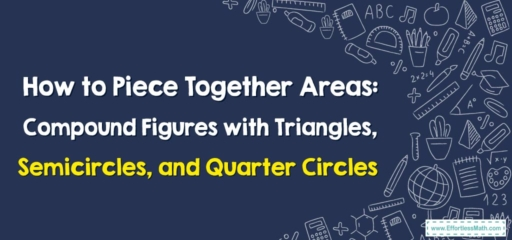# How to Piece Together Areas: Compound Figures with Triangles, Semicircles, and Quarter Circles

Hello, math enthusiasts!

Today, we're stepping into the exciting world of compound figures. These are shapes made up of more than one basic geometric figure, like triangles, circles, or semicircles. This approach will help us break down more complex shapes into manageable pieces and find their area.## 1. Understanding Compound Figures

Compound figures, also known as composite figures, consist of simple shapes combined. By identifying these simpler shapes, we can determine the area of the whole figure.

## 2. Finding the Area of Compound Figures

The method for finding the area of a compound figure involves decomposing the figure into simpler shapes, finding the areas of these simpler shapes, and then combining them.

## Step-By-Step Guide to Finding Compound Figures’ Area with Triangles, Semicircles, and Quarter Circles

Let’s break down the process:

### Step 1: Decompose the Figure

First, divide the compound figure into its constituent simple shapes. Look for triangles, semicircles, and quarter circles within the figure.

### Step 2: Find the Areas of the Simple Shapes

Next, calculate the areas of each of these shapes:

• For a triangle, the area is $$0.5\times base\times height$$.
• For a semicircle with radius r, the area is $$0.5\times π\times r^2$$.
• For a quarter circle with a radius $$r$$, the area is $$0.25\times π\times r^2$$.

### Step 3: Combine the Areas

Finally, add up all the areas to get the total area of the compound figure.

As an example, let’s say we have a compound figure made up of a triangle with a base of $$4$$ units and height of $$3$$ units, and a semicircle with a radius of $$2$$ units:

1. Decompose the figure into a triangle and a semicircle.
2. Find the area of the triangle: $$0.5\times 4\times 3 = 6$$ square units.
3. Find the area of the semicircle: $$0.5\times π\times 2^2 = 2π$$ square units.
4. Add these areas together: $$6 + 2π ≈ 12.28$$ square units.

Remember, the key is to break down the compound figure into simpler parts. This makes finding the area a manageable task.

Keep exploring, keep practicing, and enjoy your mathematical journey!

In this blog post, we’ve explained how to find the area of compound figures made up of triangles, semicircles, and quarter circles. We also provided a step-by-step guide to practice this important skill. Keep practicing, and you’ll master this useful geometric skill in no time. Happy calculating!

### What people say about "How to Piece Together Areas: Compound Figures with Triangles, Semicircles, and Quarter Circles - Effortless Math: We Help Students Learn to LOVE Mathematics"?

No one replied yet.

X
30% OFF

Limited time only!

Save Over 30%

SAVE $5 It was$16.99 now it is \$11.99